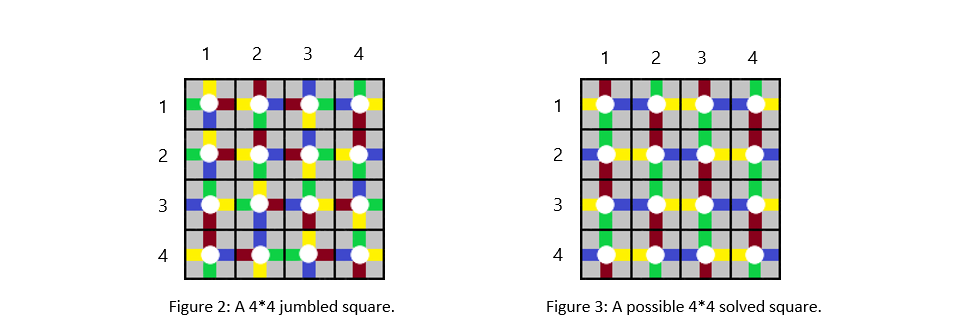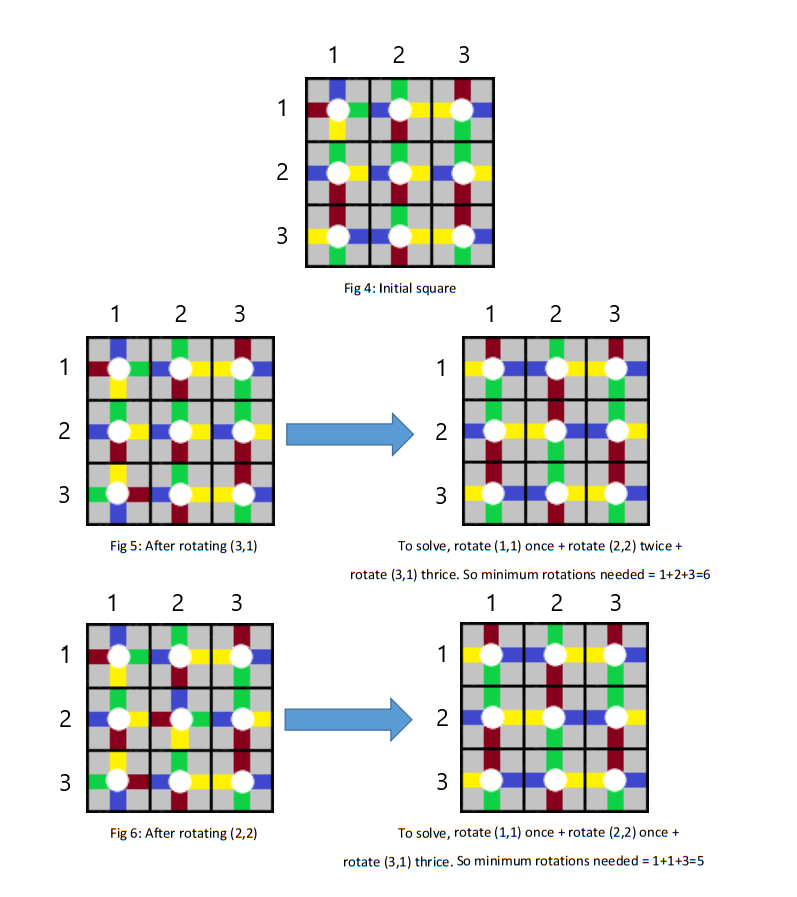# Practice on Toph

Participate in exhilarating programming contests, solve unique algorithm and data structure challenges and be a part of an awesome community.

# Robi Kaka's Cube

By Mehedi_Hassan · Limits 1s, 512 MB

Our beloved Robi Kaka has invented a cube. You have to solve only one side of this cube – a square of $N\times N$ cells. Every cell has $4$ colors – Red, Blue, Green, Yellow stripes drawn in clockwise direction one after another. There is also a white rotating button in the middle of each cell. Tapping it once rotates the cell $90°$ in clockwise direction.We define the current state of a cell with its top color. Here are the four possible states of a cell, named after their top color $–$You are given a jumbled square. Robi kaka calls it solved only if every adjacent cell of it shares same color.Being the most talented bhatija, Robi kaka will ask you $Q$ queries. Every time Robi kaka will select cell $(i, j)$ and press the white button exactly once. Then Robi kaka asks you to find the minimum number of rotations (using the white button) needed to solve the square. Every rotation made by Robi kaka takes place.

Note that, to answer each query, you just take a look at the cube and answer the minimum number of rotation required. But you do not perform any operation on the cube.

## Input

The first line contains an integer $T$ $\left(1 \leq T \leq 5\right)$, number of test cases.

For each test case the first line contains an integer $N$ $\left(1 \leq N \leq 500\right)$, length of the side of the square.

The following $N$ lines each contain a string of $N$ characters, denoting the initial square. The character in the $i$-th row and $j$-th column is one of '$\texttt{R}$', '$\texttt{Y}$', '$\texttt{G}$', '$\texttt{B}$' denoting the top color of the cell in the $i$-th row from top and $j$-th column from left of the square.

The next line will contain an integer $Q$ $\left(1 \leq Q \leq 10^5\right)$, the number of queries Robi Kaka will ask you.

The following $Q$ lines each contain $2$ numbers, $i$ and $j$ $\left(1 \leq i, j \leq N\right)$, meaning that Robi Kaka rotates the cell in $i$-th row from top and $j$-th column from left using the button in it.

## Output

For each $Q$ query, print the minimum number of rotations needed to solve the square.

## Sample

InputOutput
1
3
BGR
GGG
RGR
2
3 1
2 2

6
5•### Statistics

86% Solution Ratio

akash740Earliest, 1M ago

akash740Fastest, 0.1s

RakibJoyLightest, 3.8 MB

tahsin_protikShortest, 1549B

Login to submit

### Editorial

There are only 4 possible Solved structure/formation. Now, let's calculate and store the number of ...

### Related Contests

 CUET Intra University Junior Contest (Round 1)Ended 1M ago Replay of CUET Intra University Junior Contest (Round 1)Ended 1M ago